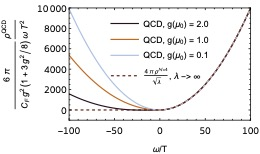## Generalized Gluon Distribution for Quarkonium Dynamics in Strongly Coupled N=4 Yang-Mills Theory

Govert Nijs, Bruno Scheihing-Hitschfeld, Xiaojun Yao |

We study the generalized gluon distribution that governs the dynamics of quarkonium inside a non-Abelian thermal plasma characterizing its dissociation and recombination rates. This gluon distribution can be written in terms of a correlation function of two chromoelectric fields connected by an adjoint Wilson line. We formulate and calculate this object in N=4 supersymmetric Yang-Mills theory at strong coupling using the AdS/CFT correspondence, allowing for a nonzero center-of-mass velocity v of the heavy quark pair relative to the medium. The effect of a moving medium on the dynamics of the heavy quark pair is described by the simple substitution T with  Sqrt[gamma]  T,  in agreement with previous calculations of other observables at strong coupling, where T is the temperature of the plasma in its rest frame, and gamma = (1 – v^2)^{-1/2} is the Lorentz boost factor. Such a velocity dependence can be important when the quarkonium momentum is larger than its mass. Contrary to general expectations for open quantum systems weakly coupled with large thermal environments, the contributions to the transition rates that are usually thought of as the leading ones in Markovian descriptions vanish in this strongly coupled plasma. This calls for new theoretical developments to assess the effects of strongly coupled non-Abelian plasmas on in-medium quarkonium dynamics. Finally, we compare our results with those from weakly coupled QCD, and find that the QCD result moves toward the N=4 strongly coupled result as the coupling constant is increased within the regime of applicability of perturbation theory. This behavior makes it even more pressing to develop a non-Markovian description of quarkonium in-medium dynamics.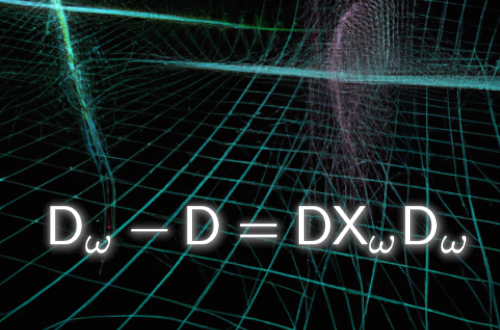## Generalized Ginsparg-Wilson relations

Hersh Singh, David Kaplan, Michael Clancy |

We give a general derivation of Ginsparg-Wilson relations for both Dirac and Majorana fermions in any dimension. These relations encode continuous and discrete chiral, parity and time reversal anomalies and will apply to the various classes of free fermion topological insulators and superconductors (in the framework of a relativistic quantum field theory in Euclidian spacetime). We show how to formulate the exact symmetries of the lattice action and the relevant index theorems for the anomalies.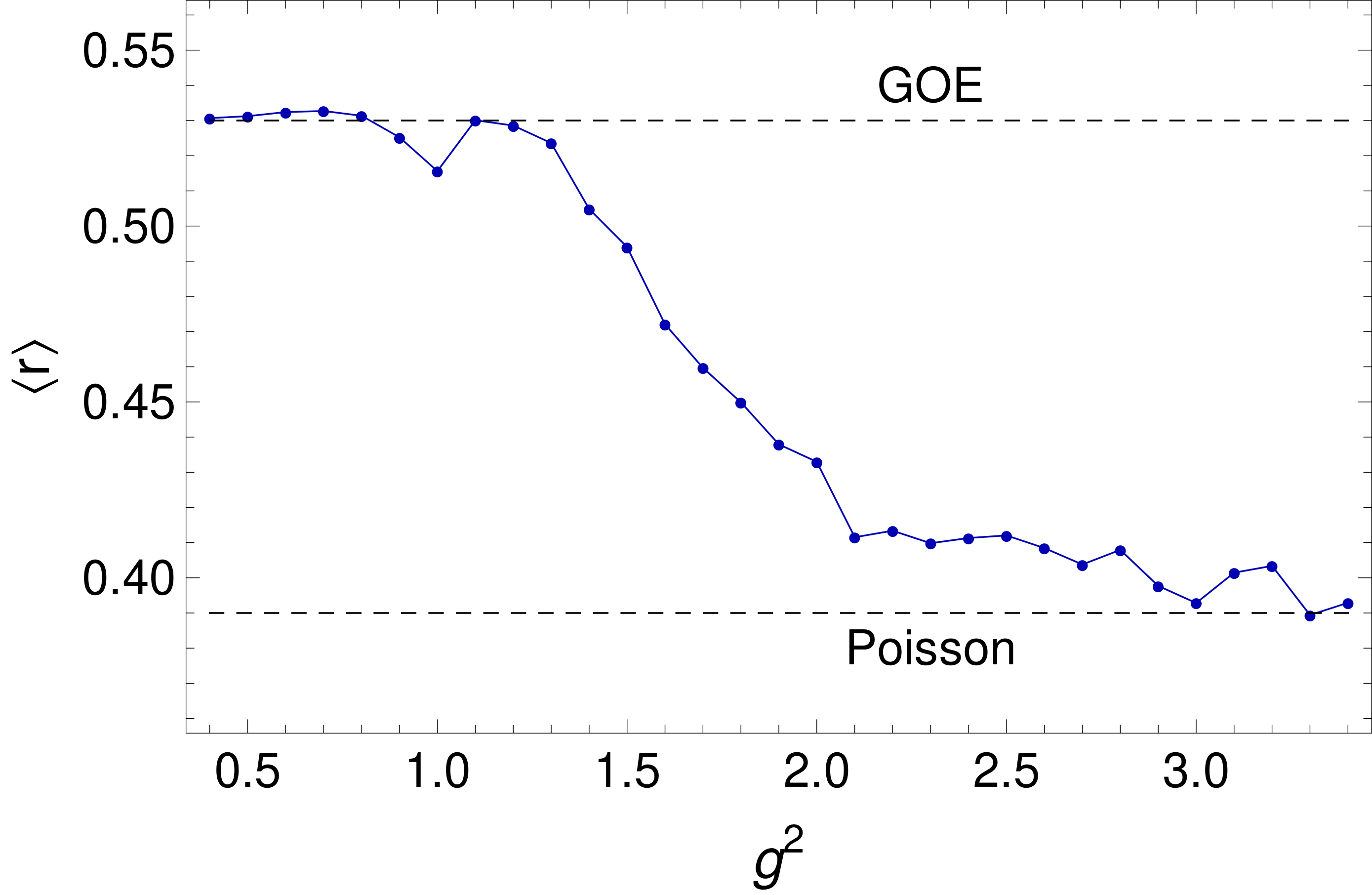## Eigenstate Thermalization in 2+1 dimensional SU(2) Lattice Gauge Theory

Lukas Ebner, Berndt Muller, Andreas Schafer, Clemens Seidl, Xiaojun Yao |

We present preliminary numerical evidence for the hypothesis that the Hamiltonian SU(2) gauge theory discretized on a lattice obeys the Eigenstate Thermalization Hypothesis (ETH). To do so we study three approximations: (a) a linear plaquette chain in a reduced Hilbert space limiting the electric field basis to j=0,1/2 , (b) a two-dimensional honeycomb lattice with periodic or closed boundary condition and the same Hilbert space constraint, and (c) a chain of only three plaquettes but such a sufficiently large electric field Hilbert space (j <= 7/2) that convergence of all energy eigenvalues in the analyzed energy window is observed. While an unconstrained Hilbert space is required to reach the continuum limit of SU(2) gauge theory, numerical resource constraints do not permit us to realize this requirement for all values of the coupling constant and large lattices. In each of the three studied cases we check first for RMT behavior and then analyse the diagonal as well as the off-diagonal matrix elements between energy eigenstates for a few operators. Within current uncertainties all results for (b) and (c) agree with ETH predictions while for case (a) deviations are found to be large for one of the analyzed observables. To unambiguously establish ETH behavior and determine for which class of operators it applies, an extension of our investigations is necessary.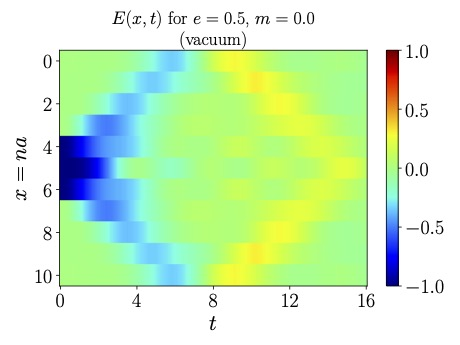## Liouvillian Dynamics of the Open Schwinger Model: String Breaking and Kinetic Dissipation in a Thermal Medium

Kyle Lee, James Mulligan, Felix Ringer, Xiaojun Yao | 2308.03878

Understanding the dynamics of bound state formation is one of the fundamental questions in confining quantum field theories such as Quantum Chromodynamics (QCD). One hadronization mechanism that has garnered significant attention is the breaking of a string initially connecting a fermion and an anti-fermion. Deepening our understanding of real-time string-breaking dynamics with simpler, lower dimensional models like the Schwinger model can improve our understanding of the hadronization process in QCD and other confining systems found in condensed matter and statistical systems. In this paper, we consider the string-breaking dynamics within the Schwinger model and investigate its modification inside a thermal medium, treating the Schwinger model as an open quantum system coupled to a thermal environment. Within the regime of weak coupling between the system and environment, the real-time evolution of the system can be described by a Lindblad evolution equation. We analyze the Liouvillian gaps of this Lindblad equation and the time dependence of the system’s von Neumann entropy. We observe that the late-time relaxation rate decreases as the environment correlation length increases. Moreover, when the environment correlation length is infinite, the system exhibits two steady states, one in each of the sectors with definite charge-conjugation-parity (CP) quantum numbers. For parameter regimes where an initial string breaks in vacuum, we observe a delay of the string breaking in the medium, due to kinetic dissipation effects. Conversely, in regimes where an initial string remains intact in vacuum time evolution, we observe string breaking (melting) in the thermal medium. We further discuss how the Liouvillian dynamics of the open Schwinger model can be simulated on quantum computers and provide an estimate of the associated Trotter errors.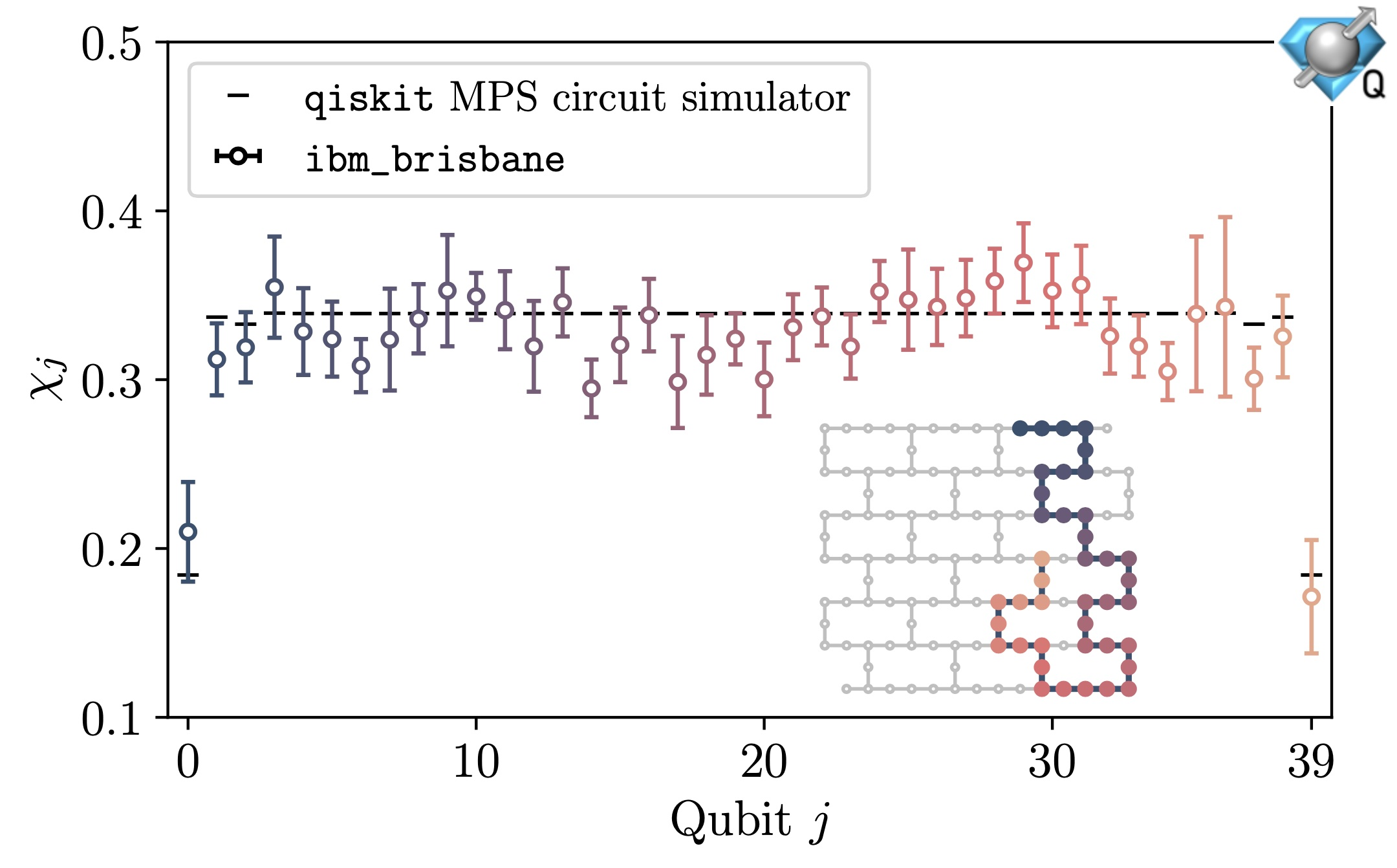## Scalable Circuits for Preparing Ground States on Digital Quantum Computers: The Schwinger Model Vacuum on 100 Qubits

Roland C. Farrell, Marc Illa, Anthony N. Ciavarella, Martin J. Savage | 2308.04481 [quant-ph]

The vacuum of the lattice Schwinger model is prepared on up to 100 qubits of IBM’s Eagle-processor quantum computers. A new algorithm to prepare the ground state of a gapped translationally-invariant system on a quantum computer is presented, which we call Scalable Circuits ADAPT-VQE (SC ADAPT-VQE). This algorithm uses the exponential decay of correlations between distant regions of the ground state, together with ADAPT-VQE, to construct quantum circuits for state preparation that can be scaled to arbitrarily large systems. SC-ADAPT-VQE is applied to the Schwinger model, and shown to be systematically improvable, with an accuracy that converges exponentially with circuit depth. Both the structure of the circuits and the deviations of prepared wavefunctions are found to become independent of the number of spatial sites, L. This allows for a controlled extrapolation of the circuits, determined using small or modest-sized systems, to arbitrarily large L. The circuits for the Schwinger model are determined on lattices up to L=14 (28 qubits) with the qiskit classical simulator, and subsequently scaled up to prepare the L=50 (100 qubits) vacuum on IBM’s 127 superconducting-qubit quantum computers ibm_brisbane and  ibm_cusco . After applying an improved error-mitigation technique, which we call Operator Decoherence Renormalization, the chiral condensate and charge-charge correlators obtained from the quantum computers are found to be in good agreement with classical Matrix Product State simulations.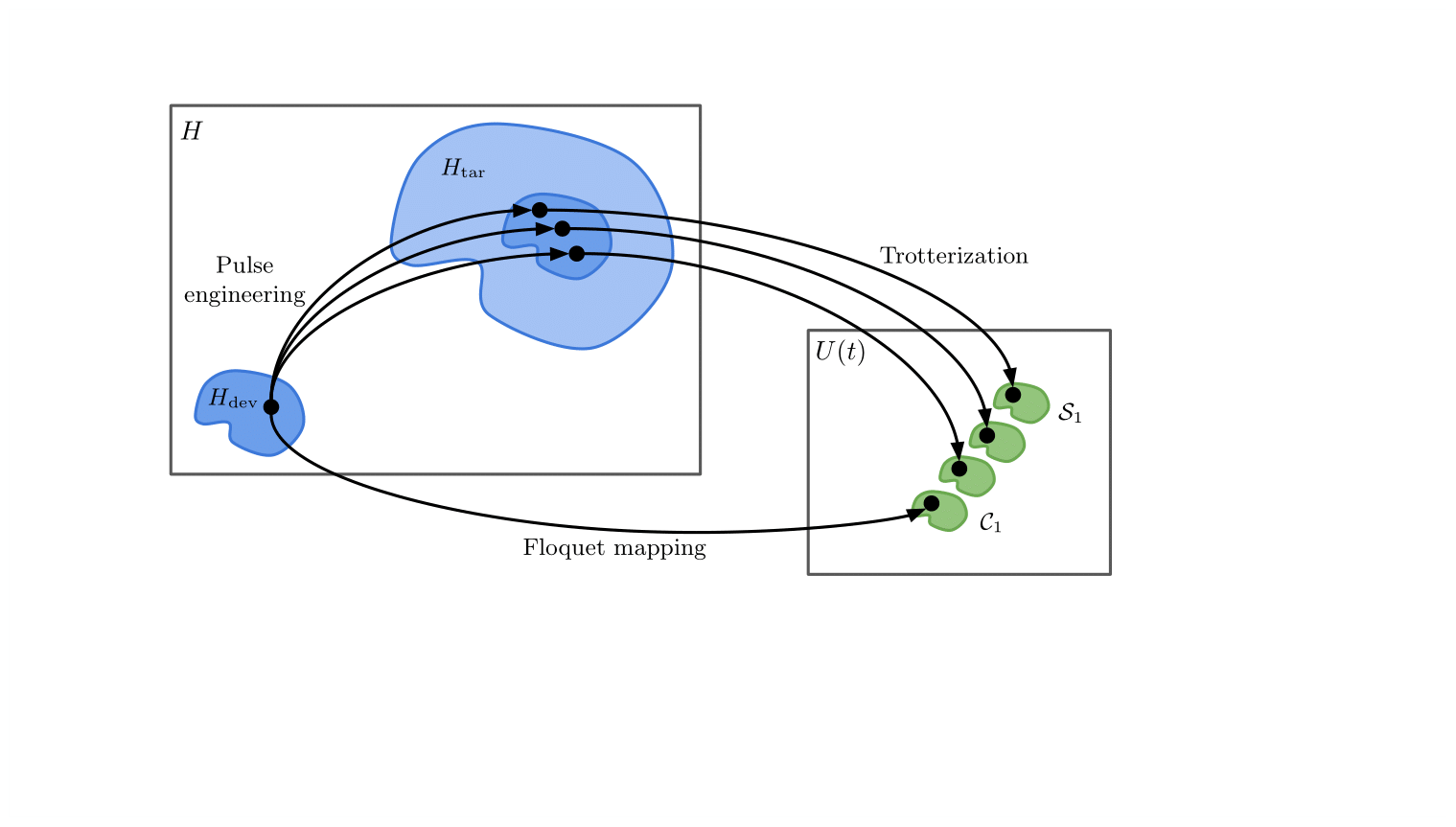## Optimization of Algorithmic Errors in Analog Quantum Simulations

Henry Froland, Stephan Caspar, Nikita Zemlevskiy | 2308.02642

Analog quantum simulation is emerging as a powerful tool for uncovering classically unreachable physics such as many-body real-time dynamics. We study a class of errors for approximate time evolution algorithms in Heisenberg-type systems on analog quantum devices described by the Ising Hamiltonian. A general framework for quantifying these errors is introduced and applied to several proposed time evolution methods, including Trotter-like methods and Floquet-engineered constant-field approaches. This analysis explains the interplay of different error sources arising from approximations to the propagator of a physical theory. The limitations placed on the accuracy of time evolution methods by current devices are discussed. Characterization of the error scaling provides a way to extend the presented Hamiltonian engineering methods to take advantage of forthcoming device capabilities.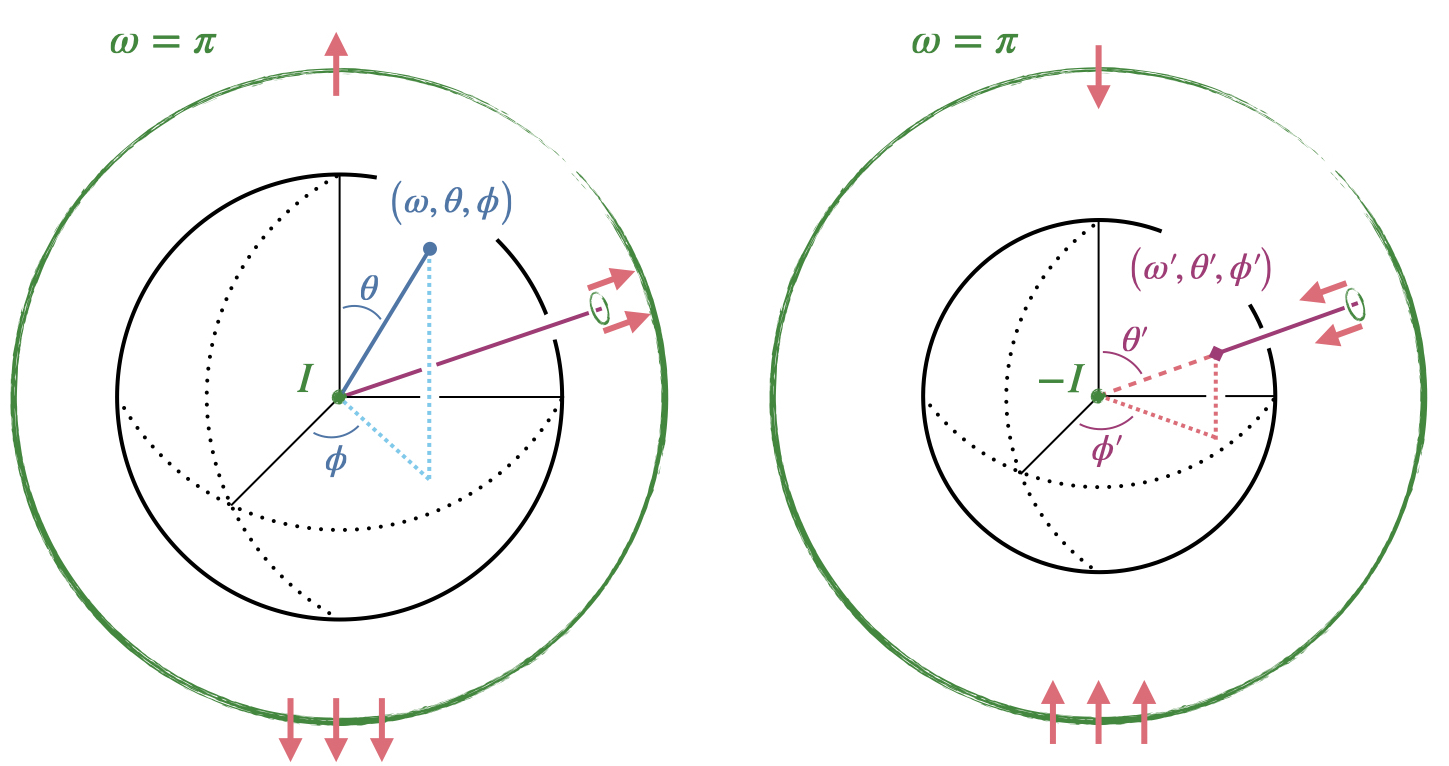## A new basis for Hamiltonian SU(2) simulations

Christian Bauer, Irian D'Andrea, Marat Freytsis, Dorota Grabowska | arxiv:2307.11829

Due to rapidly improving quantum computing hardware, Hamiltonian simulations of relativistic lattice field theories have seen a resurgence of attention. This computational tool requires turning the formally infinite-dimensional Hilbert space of the full theory into a finite-dimensional one. For gauge theories, a widely-used basis for the Hilbert space relies on the representations induced by the underlying gauge group, with a truncation that keeps only a set of the lowest dimensional representations. This works well at large bare gauge coupling, but becomes less efficient at small coupling, which is required for the continuum limit of the lattice theory. In this work, we develop a new basis suitable for the simulation of an SU(2) lattice gauge theory in the maximal tree gauge. In particular, we show how to perform a Hamiltonian truncation so that the eigenvalues of both the magnetic and electric gauge-fixed Hamiltonian are mostly preserved, which allows for this basis to be used at all values of the coupling. Little prior knowledge is assumed, so this may also be used as an introduction to the subject of Hamiltonian formulations of lattice gauge theories.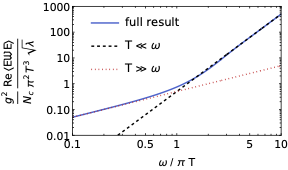## Quarkonium transport in strongly coupled plasmas

Govert Nijs, Bruno Scheihing-Hitschfeld, Xiaojun Yao | 2307.11850

Suppression of open heavy flavors and quarkonia in heavy-ion collisions is among the most informative probes of the quark-gluon plasma. Interpreting the full wealth of data obtained from the collision events requires a precise understanding of the evolution of heavy quarks and quarkonia as they propagate through the nearly thermal and strongly coupled plasma. Systematic theoretical studies of quarkonium time evolution in the QGP in the regime where the temperature of the QGP is much smaller than the inverse of quarkonium size have only been carried out in the past few years.

Such calculations require the evaluation of a gauge-invariant correlator of chromoelectric fields dressed with Wilson lines, which is similar to, but different from, the correlation used to define the well-known heavy quark diffusion coefficient. The origin of this difference has been explained previously. Here we show a calculation of the analogous correlator in strongly coupled N=4 SYM using the AdS/CFT correspondence at a finite temperature. While it resembles the open heavy quark case, it has some crucial differences that highlight the relevance of quantum color correlations. We will also discuss the results for the quarkonium transport coefficients obtained from this correlator, thereby establishing the first analytic results at strong coupling in this context.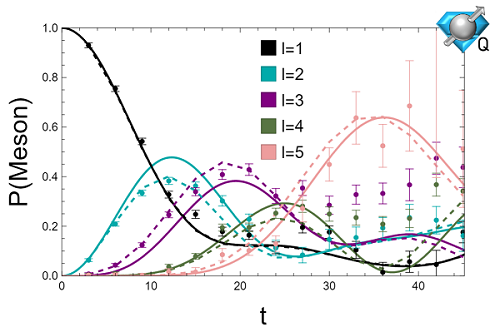## Quantum Simulation of Lattice QCD with Improved Hamiltonians

Anthony Ciavarella | arXiv:2307.05593

Quantum simulations of lattice gauge theories are anticipated to directly probe the real time dynamics of QCD, but scale unfavorably with the required truncation of the gauge fields. Improved Hamiltonians are derived to correct for the effects of gauge field truncations on the SU(3) Kogut-Susskind Hamiltonian. It is shown in 1+1D that this enables low chromo-electric field truncations to quantitatively reproduce features of the untruncated theory over a range of couplings and quark masses. In 3+1D, an improved Hamiltonian is derived for lattice QCD with staggered massless fermions. It is shown in the strong coupling limit that the spectrum qualitatively reproduces aspects of two flavor QCD and simulations of a small system are performed on IBM’s Perth quantum processor.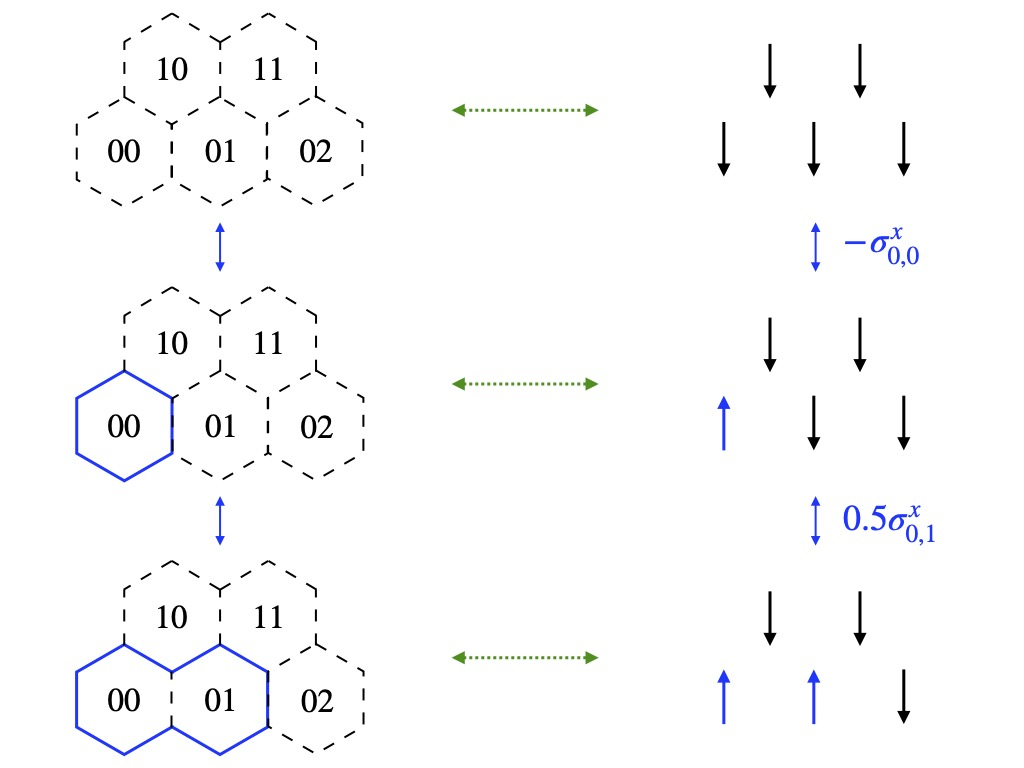## Simple Hamiltonian for Quantum Simulation of Strongly Coupled 2+1D SU(2) Lattice Gauge Theory on a Honeycomb Lattice

Berndt Mueller, Xiaojun Yao | arXiv:2307.00045

We find a simple spin Hamiltonian to describe physical states of 2+1D SU(2) lattice gauge theory on a honeycomb lattice with a truncation of the electric field representation at j_max=1/2. The simple spin Hamiltonian only contains local products of Pauli matrices, even though Gauss’s law has been completely integrated out.